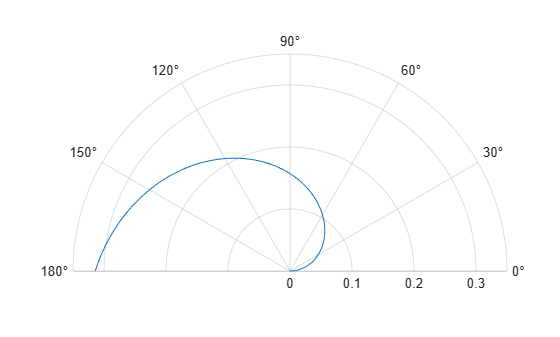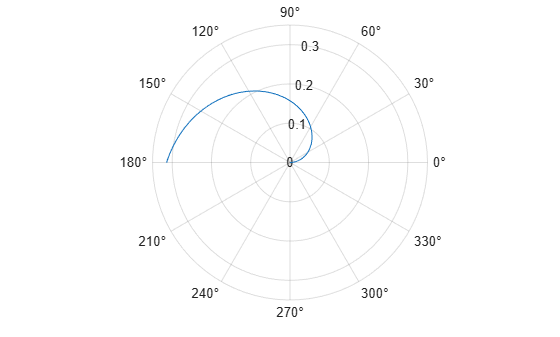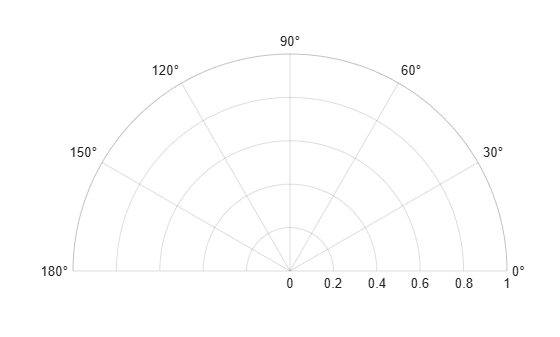Main Content

# thetalim

Set or query theta-axis limits for polar axes

## Syntax

``thetalim(limits)``
``thetalim('auto')``
``thetalim('manual')``
``tl = thetalim``
``m = thetalim('mode')``
``___ = thetalim(pax,___)``

## Description

example

````thetalim(limits)` specifies the theta-axis limits for the current polar axes. Specify `limits` as a two-element vector of the form `[thetamin thetamax]`, where `thetamax` is a numeric value greater than `thetamin`.```

example

````thetalim('auto')` lets MATLAB® choose the theta-axis limits. This command sets the `ThetaLimMode` property for the polar axes object to `'auto'`.```
````thetalim('manual')` prevents the limits from changing automatically. This command sets the `ThetaLimMode` property for the polar axes object to `'manual'`.```
````tl = thetalim` returns a two-element vector containing the limits for the current polar axes.```
````m = thetalim('mode')` returns the current value of the limits mode, which is either `'auto'` or `'manual'`. By default, the mode is automatic unless you specify limits or set the mode to manual.```

example

````___ = thetalim(pax,___)` uses the polar axes object specified by `pax` instead of the current polar axes. Specify `pax` as the first input argument. Include additional input or output arguments only if the original syntax supported them.```

## Examples

collapse all

Create a polar plot and change the theta-axis limits.

```theta = linspace(0,pi); rho = theta/10; polarplot(theta,rho) thetalim([0 180])```Set the limits back to the original values.

`thetalim('auto')`Set the limits for a specific polar axes by specifying the polar axes object as the first input to `thetalim`. Otherwise, `thetalim` sets the limits for the current axes.

```pax = polaraxes; thetalim(pax,[0 180])```## Input Arguments

collapse all

Minimum and maximum limits, specified as a two-element vector of the form ```[thetamin thetamax]```. When you specify the limits, the `ThetaLim` property for the polar axes object updates to the specified values.

Example: `[0 1]`

Polar axes, or an array of polar axes. If you do not specify this argument, then `thetalim` modifies the current axes (provided that the current axes is a polar axes object).

## Output Arguments

collapse all

Current limits, returned as a two-element vector of the form ```[thetamin thetamax]```. Querying the limits returns the value of the `ThetaLim` property for the polar axes object.

Current limits mode, returned as one of these values:

• `'auto'` — Limits automatically update to reflect changes in the data.

• `'manual'` — Limits do not automatically update.

Querying the theta-axis limits mode returns the value of the `ThetaLimMode` property for the polar axes object.

## See Also

### Properties

Introduced in R2016a

Download ebook